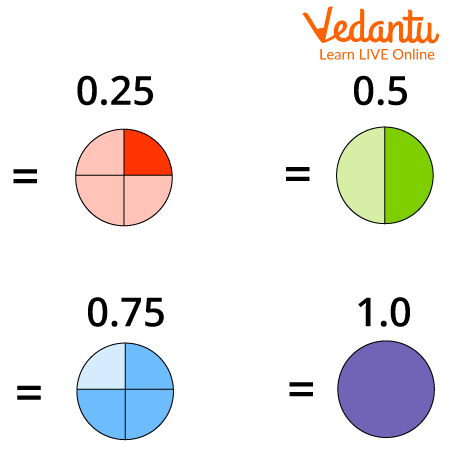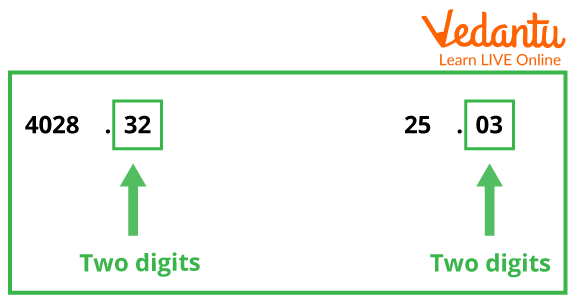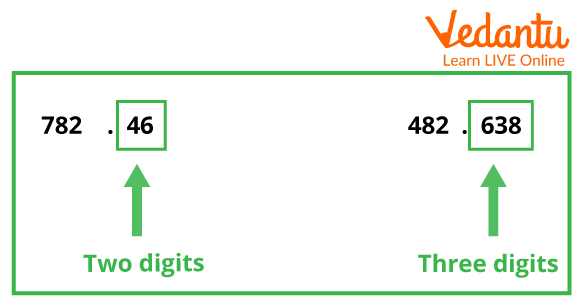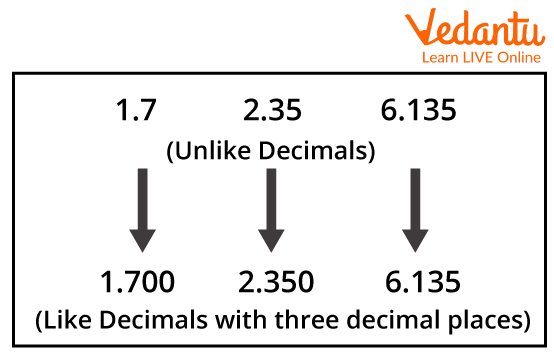Courses
Courses for Kids
Free study material
Free LIVE classes
More

# Like DecimalsLIVE
Join Vedantu’s FREE Mastercalss

## What are Like Decimals?

One of the number types in algebra with a whole integer and a fractional portion separated by a decimal point is a decimal. The decimal point is the dot between the parts of a whole number and a fraction. An example of a decimal number is 34.5. In this case, the whole number part is 34, and the fractional part is 5.

## What are Decimal Components?

A decimal number is a number in which two sets of numbers are separated by a decimal.

A decimal number has two components: an integer part and a fractional part. On the left side of the decimal, it is the integer part and on the right side of the decimal, it is the fractional part.

Integers are those values of the whole numbers which can be a positive or negative numbers.Decimals

## Types of Decimals

Decimals are of two types which are discussed below:

1. Like decimals

2. Unlike decimals

### Like Decimals:

1. Like Decimals Definition

Two decimal numbers with the same number of decimal places are said to be "like" decimal numbers. For example, \$4.12\$ and \$1.55\$ have two decimal places, so they are Like decimal.Like Decimals

• Unlike Decimal: Two or more decimal numbers are said to be unlike decimal numbers if they have a dissimilar number of digits after the decimal point. For example, \$2.6\$ and \$4.55\$ are not Like decimals; they are unlike decimals because they have different numbers of decimal places.Unlike Decimals

## What are Like Decimals?

A decimal or set of decimals or decimals with the same decimal places are similar decimals. That is, if all decimal places in a set of decimals have the same number of digits to the right of the decimal point, then that set of numbers is said to be decimal-like.Like Decimal

## Properties of Like Decimals

• Like decimals have an equal number of digits after decimals.

• We can get like decimal from unlike decimal.

• Digits after the decimal point like decimal might not be the same, they can be different.

## How to Convert Unlike Decimals to Like Decimals?

• Find the decimal point with the most decimal places.

• Now, we will add zeros to the right of the last digit of the fractional part to form another specified number of decimal places with the same number of decimal places as the highest number of decimal places.

• You need to do this because adding zeros to the right of the decimal places doesn't change the decimal value.Converting Unlike to Like Decimal

### Solved Examples

1. Check whether the following decimal is like decimals or unlike decimals.

(i) 2.45 and 6.2345

(ii) 2.34 and 2.55

(iii) 4.12 and 6.43

(iv) 3.12 and 2.111

(v) 2.90 and 1.11111

Solution:

i) 2.45 and 6.2345

The above-given decimal 2.45 has two decimal places and 6.2345 has four decimal places. Since both decimals do not have the same decimal places, they are unlike decimals.

ii ) 2.34 and 2.55

Both decimals have the same digit after decimal places, so they are like decimals.

iii) 4.12 and 6.43

Like decimals as the same number of digits after the decimal.

iv) 3.12 and 2.111

Unlike decimals, they do not have the same number of digits after the decimal.

v) 2.90 and 1.11111,

Unlike decimals, both decimals have different numbers of digits.

2. Give some examples of like decimals and unlike decimals.

Answer: Some Examples of like decimals are 3.65, 9.53, and 6.42.

Some Examples of unlike decimals are 3.76, 9.0888, and 1.2.

## Conclusion

In this article, we learned that decimals are numbers that lie between integers. They are simply another way to represent fractions. We can write more precise values of measurable quantities such as length, weight, distance, money, etc using decimals. The numbers to the left of the decimal point are integers or whole numbers, while the numbers to the right are decimal fractions.

Last updated date: 25th Sep 2023
Total views: 94.5k
Views today: 2.94k

## FAQs on Like Decimals

1. Why are decimals important?

We use decimals all the time while dealing with money, weight, length, and so on. Decimal numbers are used when greater precision is required than whole numbers provide. For example, if we calculate our weight on the weighing machine, we may only sometimes find the weight equivalent to a whole number on the weighing scale. In such conditions, the decimal system helps us to get precise values.

2. Where do we use decimals in everyday life?

When dealing with money, the usage of decimals is important. Whenever there is a purchase or sale, decimals are used. The price of products is not always expressed in whole numbers. In such situations, you'll need to know how to understand and add the given values. So, decimal knowledge is required. Decimals are also used to measure the length, weight, etc.

3. How does multiplication work when multiplying two numbers, both of which have decimal places?

Multiplication is essentially compounding two numbers to find out the resultant. So, for instance, if you received two candies per day for two days, then the results would be 3 x 3 = 9. In the case of decimal numbers, the product of the two numbers and its subsequent decimal point in the result would be based on how many decimal places the two multiplying numbers have. So if ‘9.2’ and ‘3.7’ is multiplied, then the result would be 34.04. You will see that the two multiplying numbers each have one place in decimal, and thus, as a result, the product would be two places in decimal.